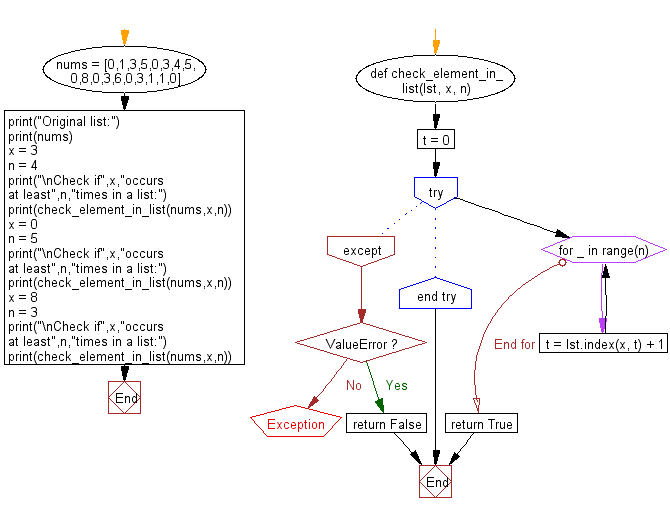﻿ Python: Check if a given element occurs at least n times in a list - w3resource# Python: Check if a given element occurs at least n times in a list

## Python List: Exercise - 153 with Solution

Write a Python program to check if a given element occurs at least n times in a list.

Sample Solution:

Python Code:

``````def check_element_in_list(lst, x, n):
t = 0
try:
for _ in range(n):
t = lst.index(x, t) + 1
return True
except ValueError:
return False

nums = [0,1,3,5,0,3,4,5,0,8,0,3,6,0,3,1,1,0]
print("Original list:")
print(nums)
x = 3
n = 4
print("\nCheck if",x,"occurs at least",n,"times in a list:")
print(check_element_in_list(nums,x,n))
x = 0
n = 5
print("\nCheck if",x,"occurs at least",n,"times in a list:")
print(check_element_in_list(nums,x,n))
x = 8
n = 3
print("\nCheck if",x,"occurs at least",n,"times in a list:")
print(check_element_in_list(nums,x,n))
```
```

Sample Output:

```Original list:
[0, 1, 3, 5, 0, 3, 4, 5, 0, 8, 0, 3, 6, 0, 3, 1, 1, 0]

Check if 3 occurs at least 4 times in a list:
True

Check if 0 occurs at least 5 times in a list:
True

Check if 8 occurs at least 3 times in a list:
False
```

Flowchart:## Visualize Python code execution:

The following tool visualize what the computer is doing step-by-step as it executes the said program:

Python Code Editor:

Have another way to solve this solution? Contribute your code (and comments) through Disqus.

What is the difficulty level of this exercise?

Test your Python skills with w3resource's quiz

﻿

## Python: Tips of the Day

Floor Division:

When we speak of division we normally mean (/) float division operator, this will give a precise result in float format with decimals.

For a rounded integer result there is (//) floor division operator in Python. Floor division will only give integer results that are round numbers.

```print(1000 // 300)
print(1000 / 300)```

Output:

```3
3.3333333333333335```